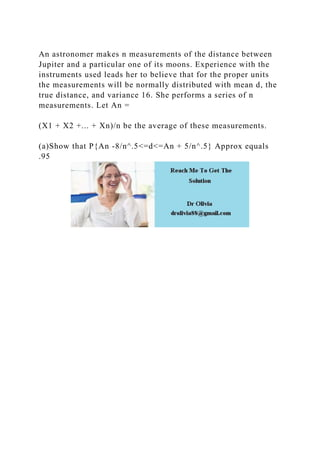Diese Präsentation wurde erfolgreich gemeldet.

# An astronomer makes n measurements of the distance between Jupiter a.docx

Anzeige
Anzeige
Anzeige
Anzeige
Anzeige
Anzeige
Anzeige
Anzeige
Anzeige
Anzeige
Anzeige×

1 von 1 Anzeige

# An astronomer makes n measurements of the distance between Jupiter a.docx

An astronomer makes n measurements of the distance between Jupiter and a particular one of its moons. Experience with the instruments used leads her to believe that for the proper units the measurements will be normally distributed with mean d, the true distance, and variance 16. She performs a series of n measurements. Let An =

(X1 + X2 +... + Xn)/n be the average of these measurements.

(a)Show that P{An -8/n^.5<=d<=An + 5/n^.5} Approx equals .95
.

An astronomer makes n measurements of the distance between Jupiter and a particular one of its moons. Experience with the instruments used leads her to believe that for the proper units the measurements will be normally distributed with mean d, the true distance, and variance 16. She performs a series of n measurements. Let An =

(X1 + X2 +... + Xn)/n be the average of these measurements.

(a)Show that P{An -8/n^.5<=d<=An + 5/n^.5} Approx equals .95
.

Anzeige
Anzeige

### An astronomer makes n measurements of the distance between Jupiter a.docx

1. 1. An astronomer makes n measurements of the distance between Jupiter and a particular one of its moons. Experience with the instruments used leads her to believe that for the proper units the measurements will be normally distributed with mean d, the true distance, and variance 16. She performs a series of n measurements. Let An = (X1 + X2 +... + Xn)/n be the average of these measurements. (a)Show that P{An -8/n^.5<=d<=An + 5/n^.5} Approx equals .95